Löwe, A., Hauptmann, M., and Majschak, J. P. (2017). "The effect of ultrasonic oscillation on the quality of 3D shapes during deep-drawing of paperboard," BioRes. 12(4), 7178-7194.

#### Abstract

In this publication, the ultrasonic-assisted deep-drawing of fiber-based materials, whose implementation was presented in Löwe et al. (2016), was studied in detail. Methods were developed for measuring the properties of deep-drawn cups, including cup stability, shape deviation, and surface quality. The relationship between these properties and the process parameters were determined with a design of experiment, which allows the user to adjust the cup properties in order to optimize them.

The Effect of Ultrasonic Oscillation on the Quality of 3D Shapes During Deep-Drawing of Paperboard

Albrecht Löwe,* Marek Hauptmann, and Jens-Peter Majschak

In this publication, the ultrasonic-assisted deep-drawing of fiber-based materials, whose implementation was presented in Löwe et al. (2016), was studied in detail. Methods were developed for measuring the properties of deep-drawn cups, including cup stability, shape deviation, and surface quality. The relationship between these properties and the process parameters were determined with a design of experiment, which allows the user to adjust the cup properties in order to optimize them.

Keywords: Paperboard; Ultrasonic vibrations; Deep-drawing

Contact information: Chair of Processing Machines and Processing Technology, Technische Universität Dresden, Bergstrasse 120, 01069 Dresden, Germany;

* Corresponding author: albrecht.loewe@tu-dresden.de

INTRODUCTION

With the depletion of fossil fuels in the near future, the packaging industry is in need of new sustainable materials. A renewable resource such as cardboard is a desirable alternative, but its possible applications are limited to objects like folded boxes, composite cans, and trays because of its low formability. Hauptmann and Majschak (2011) have studied the process of deep-drawing paperboard and achieved remarkable improvements in the quality and shape diversity of the produced cups. They found a simple manufacturing method that is easily automated and integrated into packaging lines. While Hauptmann and Majschak (2011) used heated tools to enhance the process, Löwe et al. (2016) designed ultrasonic vibrators and achieved noteworthy improvements in terms of the cup stability and surface quality. Löwe et al. (2016) designed a flexible test machine for deep-drawing, where the die vibrates in the axial and radial modes. The vibration amplitude, blank holder force, and punch velocity are continuously adjustable so that a wide range of process parameters can be studied. The publication of Löwe et al. (2016) showed how ultrasonic oscillations can be applied to deep-drawing tools. The friction between the tools and paperboard was reduced, which led to lower punch forces required during the process. It was also found that high material temperatures are reached within a short process time. However, there have been no investigations so far into how the process parameters affect the properties of deep-drawn parts. Hauptmann and Majschak (2011) explored these correlations for the conventional deep-drawing of cardboard, but the results can only be transferred partially to parts that were deep-drawn with vibrating, non-heated tools because the two methods have different temperature, friction, and compression conditions.

In this publication, the detailed results of the investigation are presented. Numerous characteristics of the cups and process (stability, surface roughness, shape deviation, consumed ultrasonic and mechanical energy) were recorded, and the effect from the process parameters was determined by a design of experiment (DoE).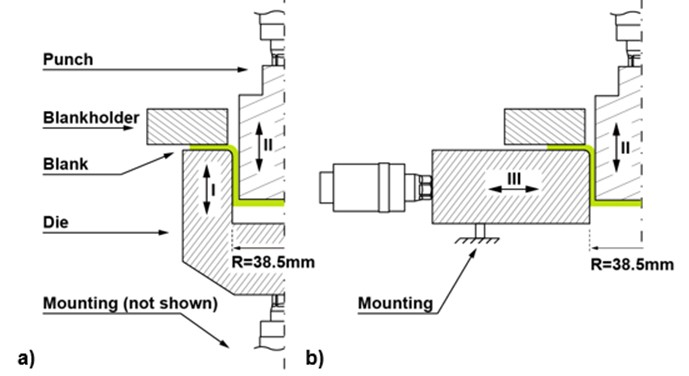Fig. 1. Cross sections of the axisymmetric tool configurations; a) axially excited die sonotrode; b) radially excited die sonotrode; I) axial vibrations of the die; II) axial vibrations of the punch; and III) radial vibrations of the die (Löwe et al. 2016)

EXPERIMENTAL SETUP

The tools and modes of vibration are shown in Fig. 1. At the start of the process, the blank is clamped firmly between the blank holder and die, and is then drawn into the cylindrical hole by the punch with a constant velocity of 10 mm/s. The blank holder force follows a linear path between its initial force (FBH) and 500 N, as was proposed by Wallmeier et al. (2015), in order to minimize the deviation of the pressure under the blank holder from its starting value.

Typical industrial carton board for microwave trays was used in the experiments (Trayforma natura (TF), STORA ENSO, Stockholm, Sweden).

Table 1. Characteristics of the Used Materials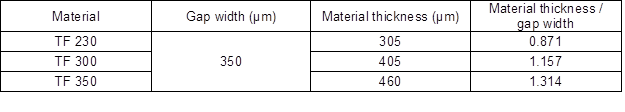The diameter of the die and punch were 77 and 76.3 mm, respectively, and the drawing gap was 350 µm. A crucial parameter for the process was the ratio between the gap width and material thickness, which was adjusted via the material thickness. A summary of the material characteristics and geometric conditions is given in Table 1.

The moisture content of the material was an important process parameter, which was adjusted in a climate chamber (Cavallo, Model C2TAI, Milano, Italy). The temperature and humidity ranged from 20 to 40 °C and 50% to 85%, respectively, with an accuracy of one decimal place, which correlated to moisture contents that ranged from approximately 7 to 12 wt.%. The weights of the conditioned and kiln-dried materials were measured on a precision scale (Kern PCB 1600-2, Balingen-Frommern, Germany) to determine the moisture content. The laboratory in which the tests were conducted had standard climatic conditions (23 °C, 50% humidity).

Löwe et al. (2016) found that a vibrating punch is rather disadvantageous, which is why only the die was excited. The frequency was 20 kHz and the vibration amplitude was adjusted from 4 to 20 µm. This amplitude was measured on the side facing the blank holder (axially vibrating sonotrode) and the gap side facing the punch (radially vibrating sonotrode). All process parameters and their levels or limits are shown in Table 2, including the initial blank holder force, ultrasonic amplitude (US amplitude), moisture content, material thickness/gap width (Mat Thkns/gap width), and vibration mode. For the regression report, all of the parameters were standardized, i.e. their minimum and maximum values corresponded to -1 and 1, respectively.

Table 2. Parameters, Types, and Levels for the Experiments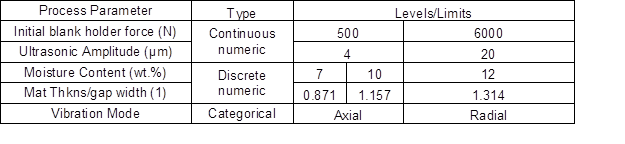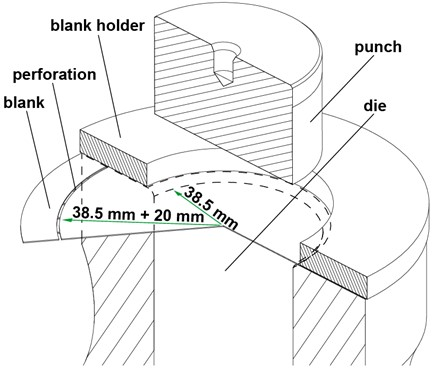Fig. 2. Isometric section view of the deep-drawing tools (axially vibrating sonotrode, punch, and blank holder)

The cups were formed with 20-mm high, straight walls without an edge on the upper side. The diameter of the blank matched the outer diameter of the sonotrode, which allowed the blank to be exactly positioned. The wall height was adjusted via the diameter of the perforated circle (Fig. 2). When the punch moved into the die, the inner part separated from the outer part, which remained under the blank holder and served as a spacer so that the tools did not touch. As soon as the inner part was fully drawn in, the vibration was turned off, and the punch and cup were pulled out.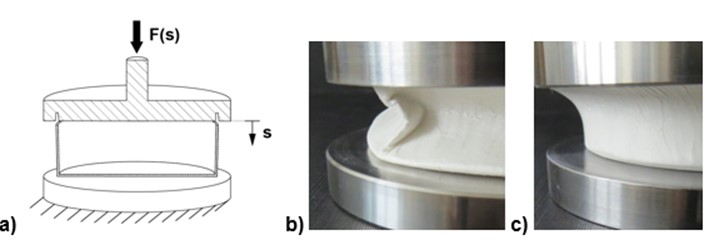Fig. 3. a) Stability test; b) buckling failure; and c) bending failure

Properties of the Cups

Stability

The stability of the cups was determined on the basis of the wall stability. A force was applied to the top edge, which was guided into a circumferential groove to ensure a consistent, cylindrical shape for every cup. This was necessary because the cups may deform to an oval shape after deep-drawing because the material is orthotropic. The groove also causes a defined buckling failure of the cup wall, so that bending failures could be eliminated during the experiments. If the edge was not guided, the walls would simply bend in some cases because the oval shape deviation leads to inclined walls, as the bottom edge has no or very little deviation from the target geometry (Fig. 3). The enforced roundness was justified because future cups will have a protruding sealing edge and may have embossing on the bottom, which forces the cups into a round shape. The wall stability was defined as the peak force of the measured curve (F(s)). This maximum force usually occurred over a relatively small distance (s) because the walls of the un-deformed cup offer the most resistance. After the highest point, damage occurs and the wall yields to the load.

Surface roughness

The surface roughness of the cup wall was defined as the Ra value (mean absolute deviation from the global mean) of the surface profile, which was measured 19 mm from the bottom of the cup (Fig. 4). A cylindrical hole was cut into the bottom of the cup to mount it onto the rotating shaft. It was held between two clamping elements, which ensured a round shape and constant distance from the confocal laser sensor (IFS 2403/90-4, microepsilon, Ortenburg, Germany). The diameter of the laser beam was only 28 µm, which ensured that even the smallest wrinkles were detected. In Fig. 5, it can be seen that the measured surface sufficiently corresponded to the real surface. The circumference of the cup was scanned five times, and the mean surface profile was calculated from these five scans in order to minimize the measurement noise.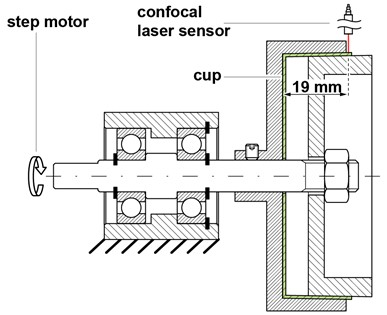Fig. 4. Setup for the surface roughness measurement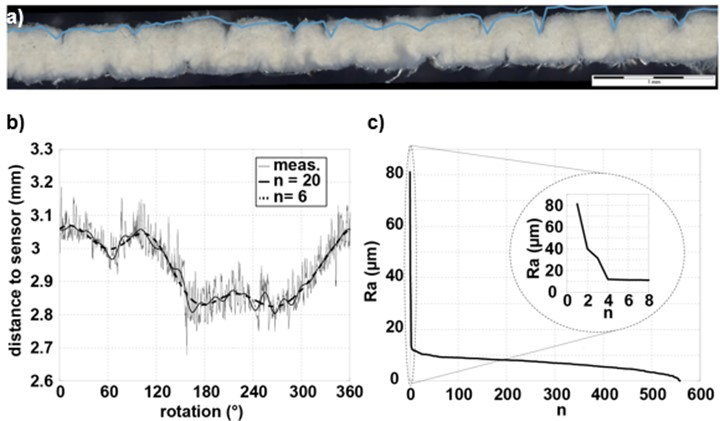Fig. 5. a) Section along the circumference of a cup overlaid with the measured surface profile; b) measured surface profile and Fourier synthesis of the data with the first n harmonics; and c) calculated Ra value as a function of the first n harmonics of the Fourier synthesis

The measured data contained not only the surface profile, but also fluctuations because of unavoidable geometrical inaccuracies of the measurement setup. Fortunately, these fluctuations were easily identified with Fourier analysis (Fig. 5b), e.g. an imbalance in the shaft led to a deviation in the signal with only one revolution along the circumference. The Fourier synthesis with the first n harmonics was therefore subtracted from the measurement data to eliminate the above-mentioned fluctuations. To determine how big n should be, a curve was calculated for every cup that showed the Ra value as a function of n (Fig. 5c). It was seen that the Ra value reached a relatively stable plateau at an n greater than 6 before slowly declining and eventually reaching 0, which meant that the Fourier synthesis with all of the harmonics was identical to the measurement data. For the evaluation of the surface roughness, an n of 6 was adopted.

Shape deviation

To assess the shape deviation, the cup was scanned with a camera system LUCIA ToolScan R360TM (Praha, Czech Republic), which scanned the surface of the cup with a laser (Fig. 6). This method was developed by Wallmeier et al. (2016). The shape deviation is defined as the average deviation of the measured points from the reference geometry, which is a cylinder in the case of round cups.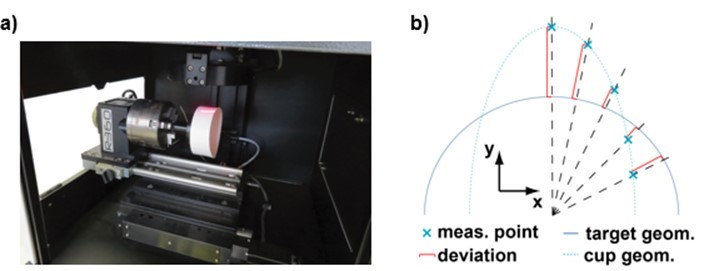Fig. 6. a) Laser scan of the cup surface; and b) calculation of the average deviation (based on (Wallmeier 2016))

Drawing depth

An experimental design was created that covered the whole parameter range. The highest drawing depth for every test point was recorded, which had at least a 70% chance of successfully producing a cup without ruptures.

Process parameters

For each deep-drawn cup, the testing machine recorded the total required mechanical and ultrasonic energy, which was calculated by integrating the force curve with respect to the punch distance and the power curve with respect to the time.

Statistical Methods

Cup properties

The collected data was subjected to a regression analysis in order to estimate the relationship between the process parameters and the properties of the deep-drawn cups, so that,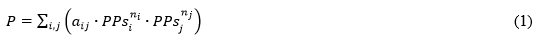where P is a property, PPs is a standardized process parameter, aij is the coefficient of regression corresponding to the product of the i-th and j-th standardized process parameters, and niand nj are the powers of the i-th and j-th standardized process parameters, respectively. The process and characteristic parameters are listed in Table. The coefficients of this model were estimated by conducting an analysis of variance (ANOVA), which calculates several test statistics to separate significant parameters from insignificant process parameters. The test statistics of the ANOVA are shown below and can be studied in detail in Chatterjee and Hadi (2006).

The R2 estimates the proportion of variation in the response that can be attributed to the model rather than to random error. The R2adj adjusts R2 for the number of parameters in the model, which enables comparisons among models with different numbers of parameters (R2adj = 1: perfect fit; R2adj = 0: the model is not a much better predictor of the response than the response mean).

1. Lack of Fit:

The Lack of Fit report gives details for a test that assesses whether the model fits the data well. The difference between the fitted and observed values can be decomposed into pure error and error because of Lack of Fit. Both errors can be used to conduct an F-test. The proposed model is an adequate approximation of the measured values if the p-value exceeds a threshold α, which was set to 0.05 for this ANOVA.

1. Estimates of the coefficients of regression:

The coefficients of regression and standard deviations were shown, along with the p-value of an F-test, which tested whether the coefficient is zero for this particular combination of coefficients of regression. The lower the p-value is, then the more likely the corresponding process parameter is significant. The parameters were standardized to the range of [-1; 1].

Probability for rupture

The characteristic parameters must be evaluable throughout the whole experimental design, e.g. in order to measure the shape deviation of a deep-drawn cup, its wall must be at least 20 mm high. However, there are parameter combinations with which a 20-mm height cannot be achieved, and therefore they must be excluded from the design. The goal was to cover the parameter range as much as possible and still have a probability of material failure of 30% at most, so that when repeating the experiment, an evaluable cup could be formed.

The probability of the occurrence of material failure in the deep-drawing of paperboard was studied in detail in Wallmeier et al. (2016) by means of logistic regression. The presented approach was followed closely for the deep-drawing of paperboard with ultrasonic assistance. The theory and application of logistic regression was described by Hosmer et al. (2013). The condition of a categorical output variable (y) (in this case: good part = 1, material failure = 0) is triggered by a function (z), which is a function of the independent variables (xi) and their estimated coefficients (bi),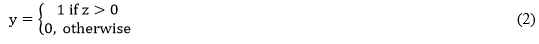And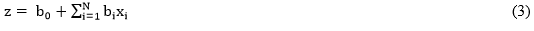The probability (p) for y to equal 1 with a certain set of independent parameters could then be calculated using Eq. 4,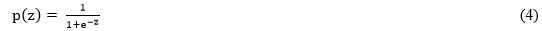The coefficients bi were subjected to a likelihood-ratio-test to determine their significance by calculating the deviation of the model, which could then be analysed with a chi-square-test. A low p-value (< 0.005) for a process parameter is a sign that it is likely to be significant in the set of independent parameters. A parameter range where p(z) was greater than 0.7 was found (i.e. a 70% chance of a successfully deep-drawn cup) and transferred to the statistical software.

Before creating an experimental design, the optimality criterion had to be chosen, which determines how the test points are distributed within the experimental space. For screening designs that focus on estimating coefficients of regression and test for significance, a D-optimal criterion is best. A detailed description of this criterion and others, like the I-optimal criterion, can be found in Goos and Jones (2011).

Examples

1. Stability (see regression report in the Appendix):

The stability was measured in newtons (N). The R2adj and Lack of Fit values were used to determine if the model with these three parameters fit the test data well. The stability was calculated with Eq. 5,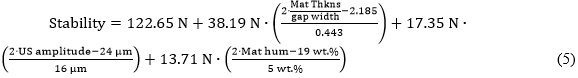The minimum achievable cup stability was 53.41 N (Mat Thkns/gap width = 0.871; US amplitude = 4 µm; material humidity (Mat hum) = 7 wt.%), and the maximum achievable cup stability was 191.9 N (Mat Thkns/gap width = 1.314; US amplitude = 20 µm; Mat hum = 12 wt.%).

1. Probability for rupture, axial sonotrode (see logistic regression report in the Appendix)

The probability for rupture with the axial sonotrode was calculated using Eq. 6,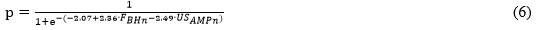where p is the probability for rupture, FBHn is the standardized blank holder force (N), and USAMPn is the standardized ultrasonic amplitude (μm).

RESULTS AND DISCUSSION

Probability for Rupture

Figures 7a and 8a show the probability of material failure when deep-drawing a 20-mm high cup with the axially and radially vibrating sonotrodes. The logistic regression reports (see ANOVA test statistics in the Appendix) showed that this probability was mainly governed by the blank holder force and ultrasonic amplitude. These results were expected because if too much blank holder force is exerted, then the friction force under the blank holder will lead to high stress in the bottom of the cup, which in turn results in failures in the material. Löwe et al. (2016) showed that the friction can be lowered with ultrasonic vibrations. This means that with a high blank holder force there must always be a sufficiently high vibration amplitude. The statistical design only included data points that were below the lines in Figs. 7b and 8b to ensure a gapless statistical design.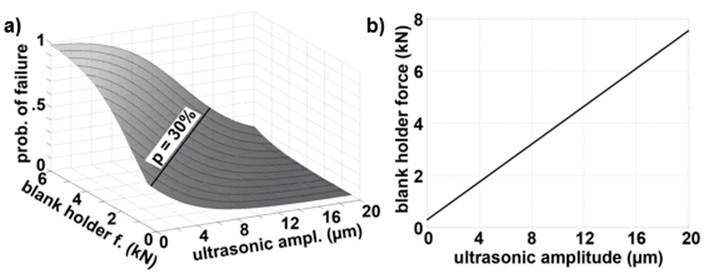Fig. 7. a) Probability of failure when deep-drawing a 20-mm cup as a function of the blank holder force and ultrasonic amplitude with the axially vibrating sonotrode; and b) linear relationship between the blank holder force and ultrasonic amplitude; the points of the statistical design must lie below the black line for a probability of material failure of less than 30%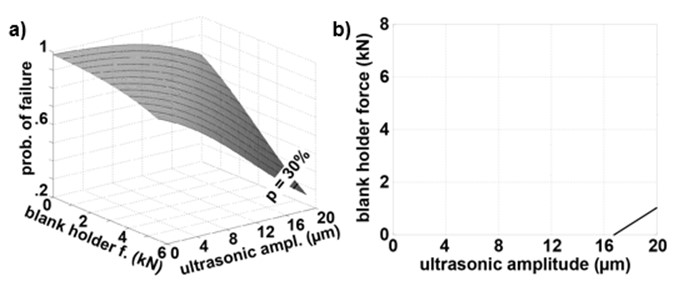Fig. 8. a) Probability of failure when deep-drawing a 20-mm cup as a function of the blank holder force and ultrasonic amplitude with the radially vibrating sonotrode; and b) linear relationship between the blank holder force and ultrasonic amplitude; the points of the statistical design must lie below the black line for a probability of material failure of less than 30%

Drawing Depth

Axial sonotrode

The dependence of the properties on the material humidity was fitted with linear and quadratic terms. Therefore, the curve was a parabola facing downward with its maximum at approximately 11.1 wt.%. The occurrence of an optimum was expected because the more humid the material is, then the higher its formability, which reduces the required punch force. This in turn reduces the stress at the bottom of the cup and allows for a greater drawing depth. A higher humidity also reduces the yield strength, which is a cause for ruptures at the bottom of the cup.

The two terms “Ultrasonic amplitude” and “Blank holder force*Ultrasonic amplitude” had a positive impact on the drawing depth. Their interaction was explained as follows. A high amplitude is always beneficial to the drawing depth, but the blank holder force both increases and decreases the height of the cup. The magnitude of the amplitude determines which of the two effects has more influence. Increasing the blank holder force leads to greater friction under the blank holder, and therefore to higher stress levels at the bottom of the cup. However, it also results in a uniform wrinkle distribution in the cup wall, whereas unevenly distributed wrinkles cause non-uniform compression in the drawing gap, resulting in stress peaks that exceed the yield strength and lead to ruptures. A high ultrasonic amplitude lowers the occurring friction, and therefore lowers the stress at the bottom of the cup. The drawing depth is lowest if there is a high blank holder force and a low ultrasonic amplitude, as was already explained in Probability for rupture.

Compression in the drawing gap, i.e. the “material thickness/gap width” ratio, is advantageous for the drawing depth because when the friction force in the gap is higher, then the force acting on the bottom of the cup is lower. If there is no compression at all, then the whole friction force under the blank holder must be absorbed by the bottom of the cup.

The experiments with the radially vibrating sonotrode had poor repeatability, which was recognizable by the low R2adj value. It was only sufficiently increased by adding the parameter “Blank holder force*Ultrasonic amplitude” to the model, the significance of which was not statistically proven. Hence, there was a low p-value for the Lack of Fit test. Another sign that the model did not fit the data adequately was the predicted negative drawing depth. The process parameters in the model were similar to the axially vibrating sonotrode parameters that were already explained. The maximum attainable drawing depth was approximately 24 mm, which was only a little higher than the required 20 mm. The radially vibrating sonotrode was not further investigated because of this, as well as the small range for successful deep-drawing. The sonotrode should be remade with titanium alloy to obtain reliable results.

Stability (Axial Sonotrode)

The stability of the cups depended primarily on the material thickness, i.e. thicker cup walls can withstand a higher axial load, which was not surprising. This was because when the material is thicker, the cross-sectional area over which the axial force is distributed is larger. Additionally, a thicker cup wall is subjected to higher compression in the drawing gap, which results in stronger interconnections between areas of the surface that are touching (Fig. 9). This effect was further enhanced by the vibration of the tools, which caused the gap to narrow periodically. A correlation between the ultrasonic amplitude and cup stability was clearly seen.

When the material was more humid, the bonds between touching parts of the surface in wrinkled areas were stronger. This conclusion confirmed the experiments of Gmeiner (2003), who patented a manufacturing method for corrugated board by joining single layers with the aid of ultrasonic vibrations. Gmeiner (2003) found that “the application of a small quantity of moisture does enhance holding power between the paper bands”.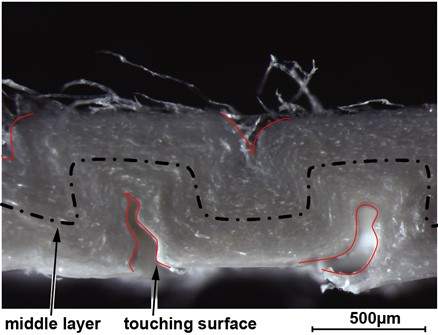Fig. 9. Cross section of a wrinkled cup wall before compression in the drawing gap

Surface Roughness (Axial Sonotrode)

The ultrasonic amplitude was the main influencing parameter on the surface roughness. A change from 4 to 20 μm reduced the Ra value by more than 30%. This basic tendency was expected because of the results reported by Wankse (2010), who found a decrease in the surface roughness during continuous roll treatment of paper webs using an ultrasound supported upper roll. The decrease in roughness was most likely because of the surface densification.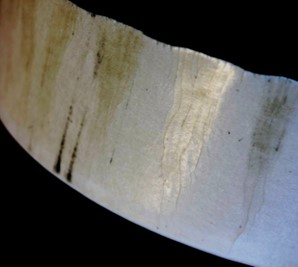Fig. 10. Close up of a cup wall that was produced with a low moisture content and blank holder force, and high ultrasonic amplitude

It does not matter how poor the wrinkle quality is because if the vibration amplitude is sufficiently large, every imperfection is smoothed. In Fig. 10, a cup is shown that was produced with a low material moisture content and low blank holder force. The wrinkles were therefore relatively large and unevenly distributed, but because of a high ultrasonic amplitude, a smooth and light reflecting surface was achieved nonetheless.

The measurements were taken at a height of 19 mm near the edge of the cup. Below that, where the material was not as thick as on the edge, the wrinkles and fiber structure were easily visible (Fig. 11a). The wall surface near the edge did not allow for the identification of single fibers and the wrinkles appeared as thin lines (Fig. 11b), which corresponded to small Ravalues. Moreover, the surface structure of the tools was engraved onto the surface of the wall. This meant that the surface roughness was strongly influenced by the compression in the drawing gap, which increased with larger drawing depths.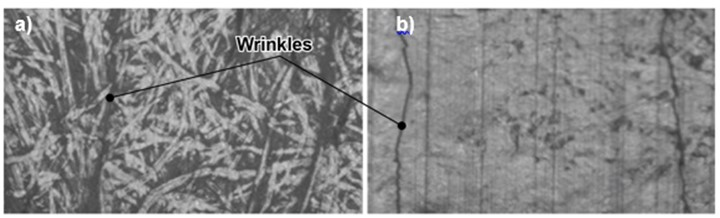Fig. 11. Light-microscopic images of the surface (a) near the bottom of the wall and (b) near the edge of the cup

Shape Deviation (Axial Sonotrode)

The shape deviation was quite large throughout the entire experimental design because there were no stiffening elements either in the bottom (embossing) or on the upper edge (sealing edge) of the cup. Therefore, the cups always had an oval shape because the material is anisotropic. The influences of the process parameters were small compared with the intercept, but were still statistically detectable.

As the ultrasonic amplitude increases so does the compaction of the material in the drawing gap, and stronger bonds are created between the touching surfaces in wrinkled areas. As a result, the cups retain their shape to a greater degree after demolding.

The blank holder force simultaneously has an increasing and decreasing effect on the shape deviation. Depending on how thick the material is, one or the other effect prevails. The shape deviation is influenced by the type of the wrinkles. The dimensional stability is higher with small, homogenously distributed wrinkles than with bigger, less homogenously distributed wrinkles. The size and distribution of wrinkles are controlled by the blank holder force. The greater the force is, the smaller and more uniform will be the wrinkles. In this study, the blank holder force decreased the shape deviation. Wallmeier et al. (2014) however found it also gave rise to higher shape deviation, as is explained in Fig. 12.

By bending the material, a tensile stress and compressive stress is induced on the punch and die sides, respectively. If the curvature is big enough, the strain will overstep the boundary to plastic behavior and a deformation will remain after relieving the load. The blank holder force causes friction and induces a tensile stress because of the punch moving downwards. This stress is superimposed with the bending stresses (Fig. 12b), which increases the ratio of plastic deformation on the punch side to that on the die side. This effect increases the shape deviation. The difference between the radii of the punch and die side is smaller with thinner materials, and thus the increasing influence of the blank holder force on the shape deviation is not as distinct as it is with thicker materials.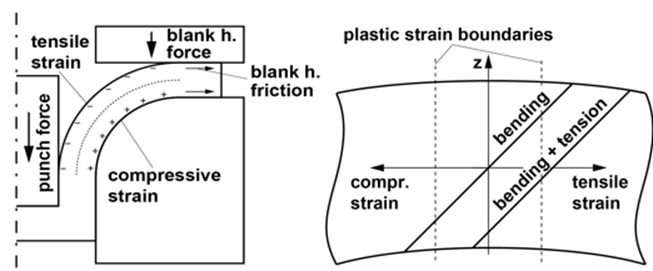Fig. 12. a) Simplified relationship between the forces and strains while deep drawing; and b) strains in the thickness direction in the bent material

Process Parameters

Ultrasonic energy (axial sonotrode)

The required ultrasonic energy is of course primarily governed by the ultrasonic amplitude, but the material thickness also has a noticeable impact. The cardboard is compacted in the drawing gap and thinner materials require less ultrasonic power. The influence of the blank holder force is not detectable. In theory, it increases and decreases the required ultrasonic energy, and these two effects would cancel out.

The blank holder presses on the upper face of the die, which moves cyclically up and down. For one half of the oscillation period, the die moves against the direction of the blank holder force, and for the other half of the oscillation period, the die moves with it. The work done is theoretically 0 J, assuming a constant amplitude and force, but in reality, no feed-in to the vibration generator is possible. Thus, the generator must provide more power when the blank holder force increases in order to maintain the vibration of the die sonotrode at the desired frequency and amplitude against the external load (Löwe et al. 2016). In contrast, if the force is higher, then the occurring wrinkles and thickness of the wrinkled material are smaller. This decreases the required punch force and ultrasonic power.

The model predicted that the minimum ultrasonic energy was in the negative range, which indicated there was a strong variation in the data (see also standard deviations of up to 32% of the measured value). This was because the ultrasonic generator always delivered just enough power so that the oscillation amplitude of the sonotrode remained constant. However, the load which the sonotrode had during deep drawing was strongly influenced by the wrinkling of the material, which was subject to large deviations.

Drawing energy (axial sonotrode)

The drawing energy depended strongly on the wrinkles in the material, which can only be partially predicted. The Lack of Fit was correspondingly poor. It only cleared the threshold of statistical significance after adding the blank holder force parameter. The punch force and drawing energy rose with a higher material thickness because of the stronger compaction and friction in the drawing gap. The ultrasonic amplitude lowered the punch force, as was reported by Löwe et al. (2016).

CONCLUSIONS

1. Analytical formulas were derived to determine the dependence of the deep-drawn paper cup properties on the process parameters. The formulas give an insight into the physical processes, allow the user to adjust the properties accurately, and furthermore, allow for optimization of the properties.
2. All of the measured cup properties benefited from a high ultrasonic amplitude, which in turn required high ultrasonic power and tended to blacken the material if the contact time between the tools and cup was too long.
3. Titanium was preferable over aluminium for the sonotrode material because the latter has a worse frictional behavior and smaller Young’s modulus. This means that the sonotrode design must be heavier when made with aluminium so that the sonotrode can withstand the process loads.
4. The probability for rupture increased with higher blank holder force because of higher stresses on the bottom of the cup. This effect can be countered with a high ultrasonic amplitude, which lowers the friction forces, and therefore the strain, on the cup.
5. If a high stability and small wrinkles are desired, then the cup will have an increased shape deviation.
6. The ultrasonic vibrations lowered the friction that occurred between the tools and material, as well as the required punch force. Thinner materials required less punch force than thicker materials.

ACKNOWLEDGEMENTS

The authors acknowledge support by the German Research Foundation and the Open Access Publication Funds of the TU Dresden

REFERENCES CITED

Chatterjee, S., and Hadi, A. S. (2006). Regression Analysis by Example, Fouth Edition, John Wiley & Sons, Ltd., Hoboken, New Jersey.

Gmeiner, J. (2003). “Vorrichtung zum kontinuierlichen Verbinden und/oder Verfestigen von Materialbahnen mittels Ultraschall,” German Patent No. EP 1900499 A2.

Goos, P., and Jones, B. (2011). Optimal Design of Experiments: A Case Study Approach, John Wiley & Sons, Ltd., Hoboken, New Jersey.

Hauptmann, M., and Majschak, J.-P. (2011). “New quality level of packaging components from paperboard through technology improvement in 3D forming,” Packag. Technol. Sci.24(7), 419-432. DOI: 10.1002/pts.941

Hosmer, D. W., Lemeshow, S., and Sturdivant, R. X. (2013). Applied Logistic Regression, John Wiley & Sons, Ltd., Hoboken, New Jersey.

Löwe, A., Hofmann, A., and Hauptmann, M. (2016). “The use and application of ultrasonic vibrations in the 3D deformation of paper and cardboard,” J. Mater. Process. Tech. 240, 23-32. DOI: 10.1016/j.jmatprotec.2016.09.006

Wallmeier, M., Hauptmann, M., and Majschak, J.-P. (2014). “New methods for quality analysis of deep-drawn packaging components from paperboard,” Packag. Technol. Sci. 28(2), 91-100. DOI: 10.1002/pts.2091

Wallmeier, M., Hauptmann, M., and Majschak, J.-P. (2016). “The occurrence of rupture in deep-drawing of paperboard,” BioResources 11(2), 4688-4704. DOI: 10.15376/biores.11.2.4688-4704

Wallmeier, M., Linvill, E., Hauptmann, M., Majschak, J.-P., and Östlund, S. (2015). “Explicit FEM analysis of the deep drawing of paperboard,” Mech. Mater. 89, 202–215. DOI: 10.1016/j.mechmat.2015.06.014

Wankse, M. (2010). Hochleistungs-Ultraschallanwendung in der Papierindustrie – Methoden zur volumenschonenden Glättung von Oberflächen, Ph.D. Dissertation, TU Dresden, Dresden, Germany.

Article submitted: June 2, 2017; Peer review completed: July 15, 2017; Revised version received: August 2, 2017; Accepted: August 5, 2017; Published: August 16, 2017.

DOI: 10.15376/biores.12.4.7178-7194

APPENDIX

Table 1. Probability for Rupture (Axial Sonotrode)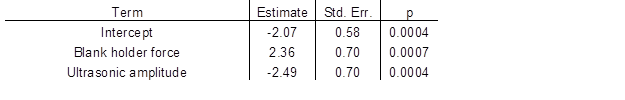R2adj = 0.54, Lack of Fit = 0.74

Table 2. Probability for Rupture (Radial Sonotrode)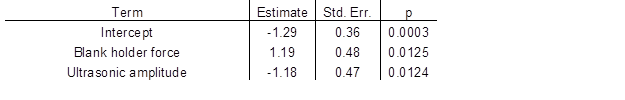R2adj = 0.19, Lack of Fit = 0.63

Table 3. Drawing Depth (mm) (Axial Sonotrode)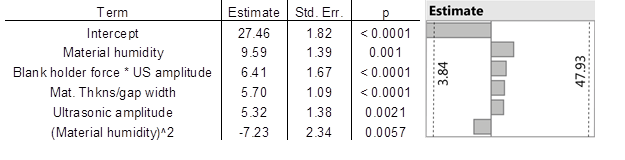R2adj = 0.87, Lack of Fit = 0.24

Table 4. Drawing Depth (mm) (Radial Sonotrode)

###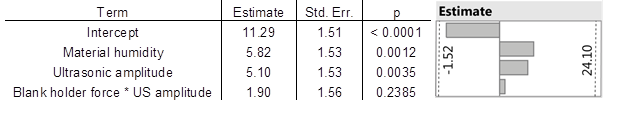R2adj = 0.90, Lack of Fit = 0.06

Table 5. Stability (N) (Axial Sonotrode)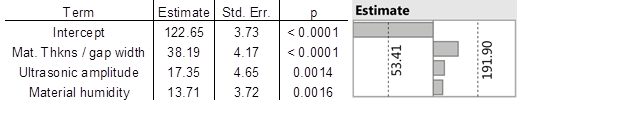R2adj = 0.85, Lack of Fit = 0.77

Table 6. Surface Roughness (µm) (Axial Sonotrode)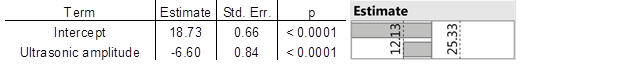R2adj = 0.75, Lack of Fit = 0.43

Table 7. Shape Deviation (mm) (Axial Sonotrode)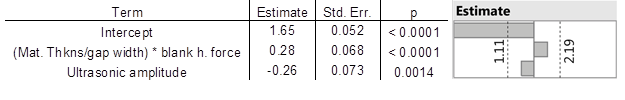R2adj = 0.51, Lack of Fit = 0.34

Table 8. Ultrasonic Energy (J) (Axial Sonotrode)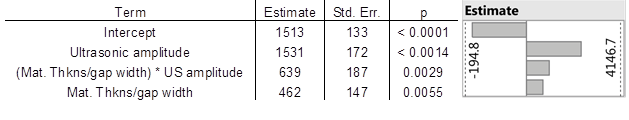R2 adj. = 0.87, Lack of Fit = 0.55

Table 9. Drawing Energy (J) (Axial Sonotrode)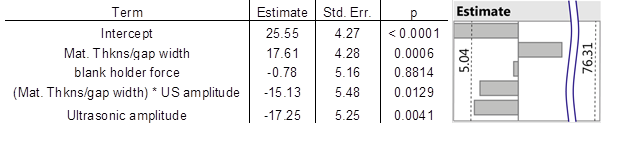R2 adj. = 0.61, Lack of Fit = 0.13